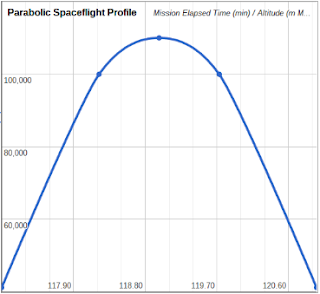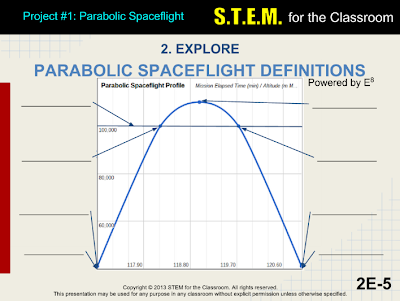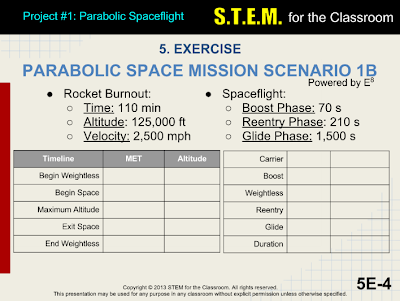## Pages

### SUBORBITAL SPACEFLIGHT

Narrative
In this, the first of four aerospace-based S.T.E.M. projects, students will calculate the Virgin Galactic SpaceShipTwo (SS2) maximum altitude, the time spent weightless, and the time spent in space.

Students will also calculate the spaceflight duration given the duration of each part of the spaceflight.

Time Frame

Mathematics Used

Material List

A connection to the Internet

Science Topics
Physics, Aerospace

11th and 12th

Essential Questions
• Who are the pioneers of parabolic spaceflight?
• What is the maximum height of a parabolic spacecraft?
• Where does space actually begin?
• When was the first COMMERCIAL parabolic spaceflight?
• Why do people want to go on a parabolic spaceflight?
• How can I be weightless if I am still increasing my altitude?
• Wait. I have to do science and technology and engineering and mathematics, all at the same time? Woah.

Activating Previous Learning
::

Lesson Overview
Note: This website incorporates spreadsheets and slide-show presentations that are provided to teachers for use in the classroom.
• Students first learn the basics of parabolic spaceflight using pencil, paper, and scientific calculator.
• Students then use what they have learned to create an Aerospace Mission Design App (AMDA), designed according to the Engineering Design Process, that will be used for real-world spacecraft. They will use spreadsheet software to create the app.
::

Learning Objectives
Evaluation
• Interpret data related to aerospace and rocketry.
• Select an optimum design from many design options to solve technological problems.
Synthesis
• Explain the principles of spaceflight in mathematical and physical terms.
• Integrate mathematics and astronautics in the engineering design process.
Analysis
• Analyze the physical principles of a parabolic spaceflight, and relate these to a space mission design.
• Use mathematics to calculate the maximum height attained by a spacecraft.
Application
• Use the Engineering Design Process to construct a real-world space mission app that is constrained by certain aerospace factors.
Comprehension
• Define constraints to the real-world model.
• Explain how solutions to the problem address the specific requirement.
Knowledge
• Explain the relationships of the principles of aerospace to the concept of maximum height.
• Demonstrate how their space mission design app addresses the requirements of maximum height.
::

Science As Inquiry
• Identify questions and concepts that guide scientific investigations.
• Design and conduct scientific investigations.
• Use technology and mathematics to improve investigations and communications.
• Formulate and revise scientific explanations and models using logic and evidence.
• Communicate and defend a scientific argument.
Physical Science
• Use mathematics and logic to explain scientific principles.
• Look up and use astronomical and astronautical constants.
Science and Technology
• Identify a problem or design an opportunity.
• Propose designs and choose between alternative solutions.
• Implement a proposed solution.
• Evaluate a solution and its consequences.
• Communicate the problem, process, and solution.
::

Visual Learning
Here is a short (3 min) video of the first Virgin Galactic SS2 rocket engine flight test. The video explains the flight testing sequence, and how it relates to eventual commercial spaceflight profiles.

2nd Flight test of the Virgin Galactic SS2 (0:02:52)

The successful test occurred 05Sep2013 at the Mojave Air and Space Port. This means that the final phase of flight testing has begun.

::

Constants
Input
• Rocket Burnout Time (min MET)
• Rocket Burnout Altitude (ft MSL)
• Rocket Burnout Velocity (mph)
Output
• Maximum Altitude (m MSL)
• Duration Spent Weightless (min)
• Duration Spent In Space (min)
::

Vocabulary
• Boost Phase: The second of six phases in a parabolic spaceflight, where the rocket engine is turned on for maximum velocity
• Begin Spaceflight: The moment a spacecraft crosses into space. Until this moment the spacecraft has been travelling in the atmosphere
• Begin Weightlessness: The moment after Rocket Burnout, when forces due to acceleration cease.
• Carrier Phase: The first of six phases in a parabolic spaceflight, where the parabolic spacecraft is carried to launch altitude
• Duration: The total time spent inside the spacecraft conducting a spaceflight
• Drop: Releasing SpaceShip 2 from the mother ship. SpaceShip 2 then falls away to a safe distance before igniting its rocket engine
• End Spaceflight: The moment a spacecraft exits from space. The spacecraft returns to the atmospheric environment
• End Weightlessness: The moment at Reentry Interface, where the spacecraft begins to slow down and gravity returns
• Glide Phase: The fifth of six phases in a parabolic spaceflight, where the spacecraft returns to the launch site in an unpowered landing
• Maximum Altitude: The highest point that a spacecraft reaches during a parabolic spaceflight
• Mean Sea Level (MSL): The distance above the average of Earth's oceans
• Mission Elapsed Time (MET): Time since the beginning of the spaceflight
• Parabolic Spaceflight: A spacecraft that coasts into space after rocket burnout that has a flight profile in the shape of a parabola
• Reentry Interface: The moment a spacecraft encounters Earth's atmosphere, which is used to slow the spacecraft down for a safe landing
• Reentry Phase: The fourth of six phases in a parabolic spaceflight, where the spacecraft comes back down into the atmosphere
• Rocket Burnout: The moment a rocket engine shuts itself off, where the spacecraft continues upward on its own momentum
• Space Interface: The height where space "officially" begins, which is set at the internationally agreed upon altitude of 100,000 m MSL, or 62 mi MSL
• SpaceShipTwo: The spacecraft that is dropped from White Knight 2. After rocket burnout, the spacecraft coasts up to space and back
• White Knight 2: The mother ship that carries SpaceShipTwo to launch altitude
• Weightless Phase: The third of six phases in a parabolic spaceflight, where the spacecraft and its occupants experience weightlessness
::

Analysis
A parabolic spacecraft, such as SS2, follows a flight profile that takes the shape of a parabola, hence the name. A parabola can be described with a quadratic equation, so that is what we will use.

The SS2 follows a similar trajectory that a baseball thrown to another baseball player follows. As all baseball players are aware, a baseball is never thrown in a straight line; rather it is thrown slightly upward. As a result, the path the baseball follows is curved (parabolic).

If the same baseball is thrown straight into the air, it will continue moving upward after it leaves the ball player's hand. The ball will eventually reach a maximum height, and then drop back down to Earth (or into the player's glove, in this case).

Note: the moment the player releases the ball, the ball is weightless. Weight returns when the ball is catched.

That baseball (spacecraft) follows a nice parabolic curve, and can be described by the following quadratic equation:
where
• h = height [m]
• a = 0.5(Standard Gravity) [m/s^2]
• v0 = Rocket Burnout Velocity [m/s] (GIVEN)
• h0 = Rocket Burnout Height [m] (GIVEN)
• t = time [s]Parabolic Spaceflight Profile
We now need to find the vertex of the parabola, which is the maximum point on the parabola. The x-component of the vertex yields the time at maximum height, and the y-component of the vertex is the maximum height.

and

where
• a = constant = 0.5(-9.8 m/s^2) = -4.9 m/s^2
• vertext = t-component of the vertex = Time at Maximum Height [s]
• vertexh = h-component of the vertex = Maximum Height [m MSL]

We now want to determine the time that we spend in space. That means letting h = 100000 and rearranging the equation so that it equals to zero:

where
• h1 = h0 - 100000

Using the Quadratic Formula, we can solve for t:
where
• t1 = Time spacecraft enters space [min MET]
• t2 = Time spacecraft exits space [min MET]

and so
Total Time In Space = t2 - t1

and

Total Time Weightless = 2 * Vertext

We now have all the information that we need to build our app.

For example:

INPUT
Rocket Burnout Time = 110 min MET
Rocket Burnout Altitude = 135,000 ft MSL
Rocket Burnout Velocity = 2,600 mph

OUTPUT
Begin Weightless
Begin Space
Maximum Altitude
End Space
End Weightless

Carrier Phase
Boost Phase (Given)
Weightless Phase
Reentry Phase (Given)
Glide Phase (Given)
Mission Duration
Total Time in Space

First, we must change our inputs to S.I. units:

Rocket Burnout Timesec = Rocket Burnout Time * 60 = 6600 s
Initial Altitude = Rocket Burnout Altitude / 3.28 = 41148 m
Initial Velocity = Rocket Burnout Velocity * 1609 / 3600 = 1162 mps

So,

Begin Weightless Time = Rocket Burnout Time = 110.00 min MET
Begin Weightless Altitude = Initial Altitude = 41148 m

Begin Space Time = Rocket Burnout Time + t1 = 111.22 min MET
Begin Space Altitude (Given) = 100000 m

Maximum Altitude Time = Rocket Burnout Time + vertext = 111.98 min MET
Maximum Altitude = vertexh = 110027 m

End Space Time = Rocket Burnout Time + t2 = 112.73 min MET
End Space Altitude (Given) = 100000 m

End Weightless Time = Rocket Burnout Time + Total Time Weightless = 113.95 min MET
End Weightless Altitude = Initial Altitude = 41148 m

and

Carrier Phase = Rocket Burnout Time - Boost Phase = 108.83 min
Boost Phase (Given) = 70 s = 1.17 min
Weightless Phase =Total Time Weightless = 3.95 min
Reentry Phase (Given) = 3.50 min
Glide Phase (Given) = 25.00 min
Mission Duration = Sum of the 5 phases above = 142.45 min
Total Time in Space = 1.51 min

The app can now be constructed and the numbers entered.

::

The Space Mission App
The Parabolic Spaceflight app is broken into four parts:
1. Input/Output
2. Graph
3. Constants
4. Calculations

Working Prototype of the Suborbital Spaceflight Spreadsheet App

::

Working Prototype of the Suborbital Spaceflight Mobile App

::

Teacher Lesson Plan
Use the slide-show presentation below to give a lesson about basic parabolic spaceflight mechanics and calculating the vertex of a parabola.

In this lesson, students will identify the various aspects of a parabolic spaceflight diagram, matching them with the terms and definitions.

Students then practice the calculations using pencil, paper, and calculator.

Students then learn about the Engineering Design Process, and begin the process of laying the ground work for the app built with a spreadsheet. Sample Open Source computer code is provided to aid students with their spreadsheet formulas.

Some screenshots of the Teacher Presentation:Vocabulary Fill-In of a Graph of the Weightless Phase of a Parabolic SpaceflightComparing Parabolic Spacecraft Then and Now...Parabolic Spaceflight Student WorksheetOpen Source Cloud Computer Code
The slides will guide the students as they run through the lesson powered by E^8:
1. Engage
• Lesson Objectives
• Lesson Goals
• Lesson Organization
2. Explore
• The Parabola and its Components and Definitions
3. Explain
• The Vertex
• The Vertex as a Maximum
• Mission Duration Equation
4. Elaborate
• Other Parabolic Spacecraft Examples
5. Exercise
• Parabolic Space Mission Parameters
• Parabolic Space Mission Design Scenario 1
• Parabolic Space Mission Design Scenario 2
6. Engineer
• The Engineering Design Process
• AMDA Spaceflight Plan
• Designing a Prototype
• AMDA Software
7. Express
• Displaying the AMDC
• Progress Report
8. Evaluate
• Post Engineering Assessment
This lesson can be delivered in one or two class periods, with students working on the project after school. It is recommended that a few minutes of a few class periods be set aside for student help.

The Student Workbook (below) accompanies the Teacher Lesson Plan.

::

Missions
The input values of the app can be varied to create different spaceflight scenarios. These scenarios will add a sense of realism to the student project. In addition, students will be placed into groups. Each group will be assigned the following positions:
• Cloud Applications Engineer
• Engineering Log
To make learning fun, each group could create a name for itself. Callsigns for the individual group members would add to the merriment.

The groups will be asked to determine the maximum height, total time weightless, and the total time in space of the passengers aboard SpaceShipTwo:

Encourage groups to design their own scenarios by using different input values. This project is very flexible in that regard.

::

Presentation
Students will be asked to present their findings to the rest of the class. Parents are, of course, encouraged to attend (it is suggested that a pot luck would make things more festive).

Each presentation will have slides that introduces the group, describes the spaceflight, and displays the calculations. A short biography of Sir Richard Branson.

Students that know how to use presentation software should be encouraged to create their own presentations (remember, Google Apps are free!)

::

Rubric
Students will also create a website and embed their slide presentation and their S.T.E.M. app in a webpage. Their journal will be kept on the webpage as well. If the class presentation is recorded to video, it can be uploaded to YouTube, then embedded in the webpage.

Therefore, each webpage (one for each project) should have the following items:
1. Embedded Slide Presentation
2. Embedded view of S.T.E.M. app
4. Link to working prototype of S.T.E.M. app
5. Journal Entries
6. (optional) Embedded YouTube video of the presentation

This is not a scoring rubric; rather it is a guide of what is expected for the project.

The presentation should take between 5 and 10 minutes, unless there are a lot of questions from the audience. For a class with 6 groups, this comes out to between 30 and 60 minutes.

Students should be encouraged to dress professionally, and to practice their presentations beforehand.

Scoring and grading these projects is left up to the professionalism of the teacher.

::

Conclusion
The quadratic equations used in this project should be easy enough for the average high school Algebra 2 student. The teacher may need to guide students through the setup of the equations and the calculations. As the quarter progress, the concepts and the mathematics will become more challenging.

Using technology to do a high school math project should be easy and fun.

But above all, it should be free.

Both the spreadsheet and the presentation were built using Google Docs, a free application when you sign up for gmail through Google. Therefore, any student with an Internet access can use these tools for free, whether at the school, or at the library, or at the coffee shop, or at home, etc.

Students that do well on this project will learn many important skills that will help to succeed in whatever field they desire to choose.

::